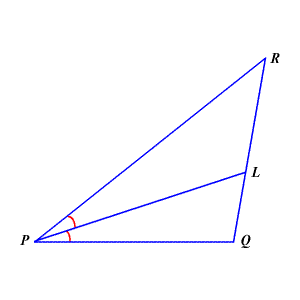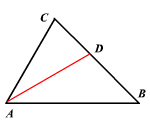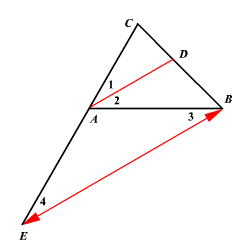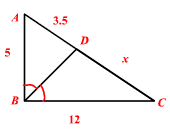# Angle Bisector Theorem

Warning: This name is used differently in different textbooks. In some textbooks, it refers to the theorem which states that any point on an angle bisector is equidistant from the two sides of the angle.

What most textbooks call the Angle Bisector Theorem is this:

An angle bisector in a triangle divides the opposite side into two segments which are in the same proportion as the other two sides of the triangle.In the figure above, $\stackrel{¯}{PL}$ bisects $\angle RPQ$ , so $\frac{RL}{LQ}=\frac{PR}{PQ}$ .

## Triangle Angle Bisector Theorem

An angle bisector of an angle of a triangle divides the opposite side in two segments that are proportional to the other two sides of the triangle.By the Angle Bisector Theorem,

$\frac{BD}{DC}=\frac{AB}{AC}$

Proof:

Draw $\stackrel{↔}{BE}\parallel \stackrel{↔}{AD}$ .

Extend $\stackrel{¯}{CA}$ to meet $\stackrel{↔}{BE}$ at point $E$ .By the Side-Splitter Theorem,

$\frac{CD}{DB}=\frac{CA}{AE}$ ---------(1)

The angles $\angle 4$ and $\angle 1$ are corresponding angles.

So, $\angle 4\cong \angle 1$

Since $\stackrel{¯}{AD}$ is a angle bisector of the angle $\angle CAB$ , $\angle 1\cong \angle 2$ .

By the Alternate Interior Angle Theorem , $\angle 2\cong \angle 3$ .

Therefore, by transitive property, $\angle 4\cong \angle 3$ .

Since the angles $\angle 3$ and $\angle 4$ are congruent , the triangle $\Delta ABE$ is an isosceles triangle with $AE=AB$ .

Replacing $AE$ by $AB$ in equation (1),

$\frac{CD}{DB}=\frac{CA}{AB}$

Example:Find the value of $x$ .

By Triangle-Angle-Bisector Theorem,

$\frac{AB}{BC}=\frac{AD}{DC}$ .

Substitute.

$\frac{5}{12}=\frac{3.5}{x}$

Cross multiply.

$5x=42$

Divide both sides by $5$ .

$\begin{array}{l}\frac{5x}{5}=\frac{42}{5}\\ \text{\hspace{0.17em}}\text{\hspace{0.17em}}x=8.4\end{array}$

The value of $x$ is $8.4$ .